# 02 SPICE

### Prof. Dr. Jörg Vollrath

01 Introduction and basic properties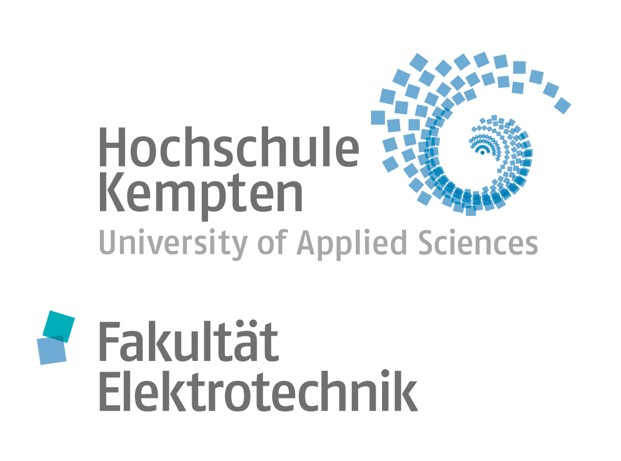## Video 2. lecture

 Video is not visible, most likely your browser does not support HTML5 video Länge: 01:06:27 0:0:0 Review Introduction 0:0:44 Video recording 0:1:34 LTSPICE start 0:2:49 LTSPICE configuration white background and thick lines 0:5:13 Thick lines 0:7:29 Start schematic with resistor 0:8:44 Finished low pass placing parts 0:12:12 Values for components 0:12:44 Labels node names 0:13:19 Simulate .op 0:14:59 Turning a resistor changes polarity of current 0:16:12 Simulate .dc voltage ramp 0:17:1 Add trace to graph 0:18:44 Sine voltage at source 0:19:44 Transient simulation .tran 0:20:30 Graph discussion 0:21:6 Higher frequency 0:22:36 AC simulation, voltage source and command 0:24:14 Graph discussion 0:25:6 Corner frequency 0:26:56 Context sensitive menues 0:27:36 Measure command .MEAS 0:28:54 Verification of result 0:30:13 Data converter schematic 4 Bit DAC 0:31:36 Copying SPICE code to local drive 0:32:36 Copying subcircuits .asc .asy 0:33:56 ADC circuit download 0:34:37 ADC DAC test circuit 0:35:33 Explanation test circuit 0:36:33 Hierarchie 0:37:18 Sample and hold 0:38:43 .Save command 0:40:13 16 steps 0:41:53 Voltage probe 0:42:23 High frequency sampling 0:44:25 12 Bit test circuit 0:45:58 Tools for data processing and analysis 0:47:28 Webreport 0:48:28 Directories and files 0:51:1 Presentation and handout mode 0:51:33 Editor 0:53:28 HTML tags 0:55:11 LTSPICE schematics from files 0:57:44 Equations with MathJax 0:58:19 Animations 0:59:53 Insert images 1:1:33 Copy, paste, modify

# Overview

 Introduction to SPICE (Circuit Simulation) Program Link Syntax Examples Hierarchy SPICE and data converters Modelling level: Transistors Behavioral (analytic expressions) Hierarchy Waveform Generation, Data Export and Measurements DAC model ADC model CMOS: Circuit Design, Layout and Simulation, Baker, Chap1, p.8-29 Video is not visible, most likely your browser does not support HTML5 video

# SPICE

 Circuit Simulator Draw Circuits Generate a netlist What is a netlist? Calculates voltage and currrents Nonlinear Elements (Transistor/Diode) Transient simulation: voltage and current over time Frequency dependent Amplitude and Phase Numerical solution LTSPICE für Windows http://www.linear.com/designtools/software/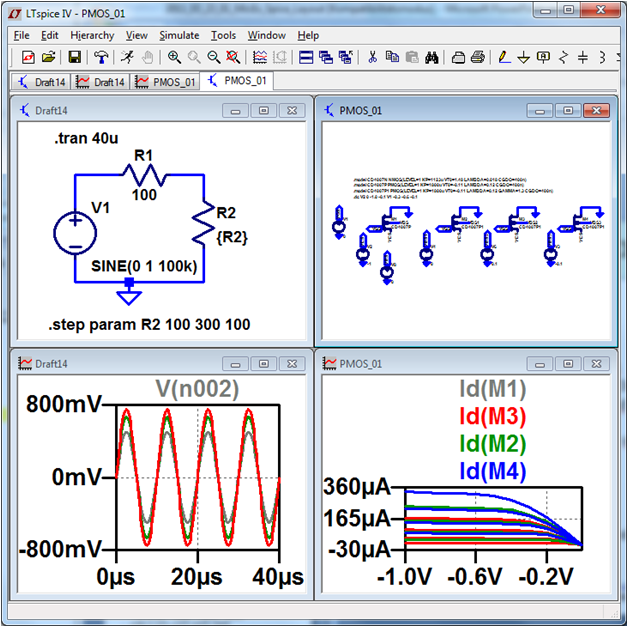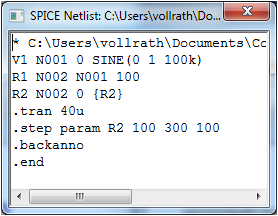# LTSPICE netlist

View, SPICE netlist

* C:\Programme\LTC\LTspiceIV\Draft1.asc
V1 N001 0 1
R1 N002 N001 50
R2 N002 0 50
.dc V1 0 2 0.1
.backanno
.end

V is a voltage source
N001, N002 node names
.dc simulation instruction

# SPICE Elements

• Voltage source
• DC voltage: V<name> <node1> <node2> <value>
• AC voltage:
SIN <offset> <amplitude> <frequency> [<delay>]
• LTSPICE: Menue Edit, Component, Voltage
• Current source
• like voltage, but starting with I
• Resistance
• R<name> <node1> <node2> <value>
• Capacitance
• C<name> <node1> <node2> <value>
• Inductance
• L<name> <node1> <node2> <value>
• Modifiers: m (10-3), u(10-6), k(103), MEG(106)

# SPICE simulation statements

• Operating point (voltage, current)
• .op
• Variation of a source
• .dc <sourcename> <startvalue> <endvalue> <step>
• Variation of multiple parameters
• .dc statement with multiple elements
• .dc VDS 0 5 1m VGS 0 5 1
• Transient simulation(time)
• .tran <tstep> <tstop> [<tstart> <tmax>]
• Transferfunction
• .TF V(<node1>,<node2>) Vin
• .TF I(<Elementname>)
• Bodeplot
• .ac <dec> <Npoints> <startfrequency> <EndFreq>

# LTSPICE: First Steps

 File, New Schematic Edit, Place Ground Edit, Component, Voltage < ESC >: Stop drawing Edit, Resistor Value: Right click on component 50 Ohm, 1V value without unit Edit, Draw Wire Simulate, Edit Simulation Command (Tab DC Sweep) 1st Source, Name V1, Start 0, Stop 2, Step 0.1 Simulate, Run Maus: right click, Add Trace Info: lower left corner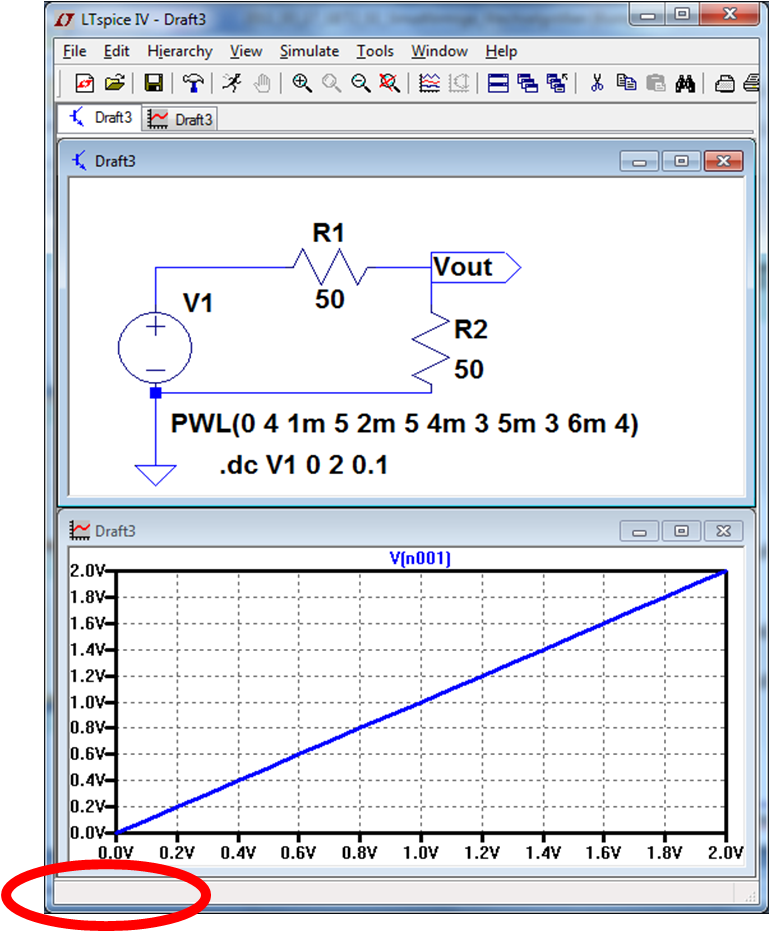# LTSPICE: Configuration

 White background Tools, Color Preferences, Background Thick lines Tools, Control Panel Waveforms plot data with thick lines Drafting options, thick lines Currents and voltages of subcircuits Tools, Control Panel, Save Defaults Caution: Generates big files on hard disc: Tools, Control Panel, Operation, Automatically delete Documentation Help Tools, Copy bitmap to clipboard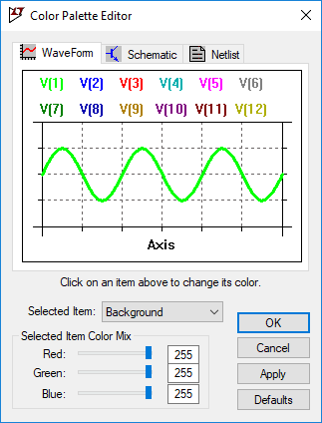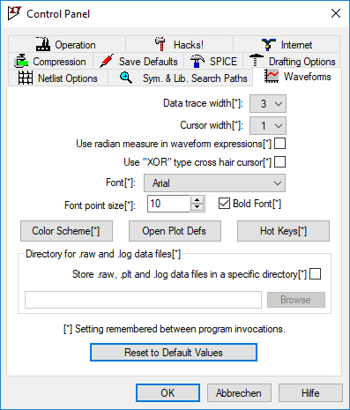# Example Voltage divider operation point: .op

 Schematic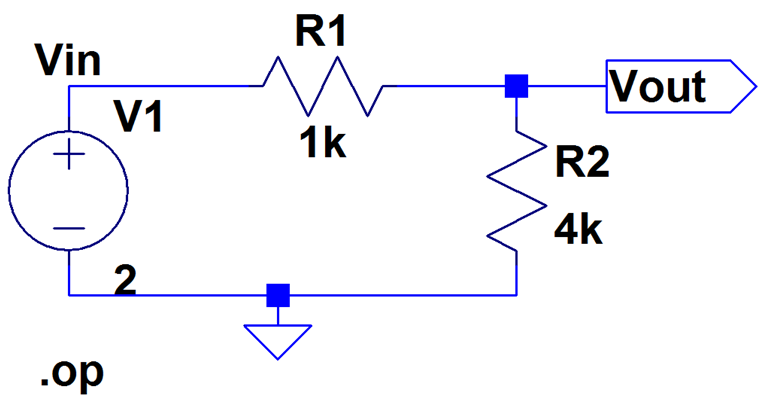Voltage source: Vin Resistor: R1, R2 (Orientation -> current) Nodes: Vin, Vout, 0 Analysis: .op operation point Modifier: k Netlist * Spannungsteiler.asc V1 Vin 0 2 R1 Vout Vin 1k R2 Vout 0 4k .op .backanno .end Output: --- Operating Point --- V(vin): 2 voltage V(vout): 1.6 voltage I(R2): 0.0004 device_current I(R1): -0.0004 device_current I(V1): -0.0004 device_current

# Voltage source variation: .dc Vin 0 1 1m

 Schematic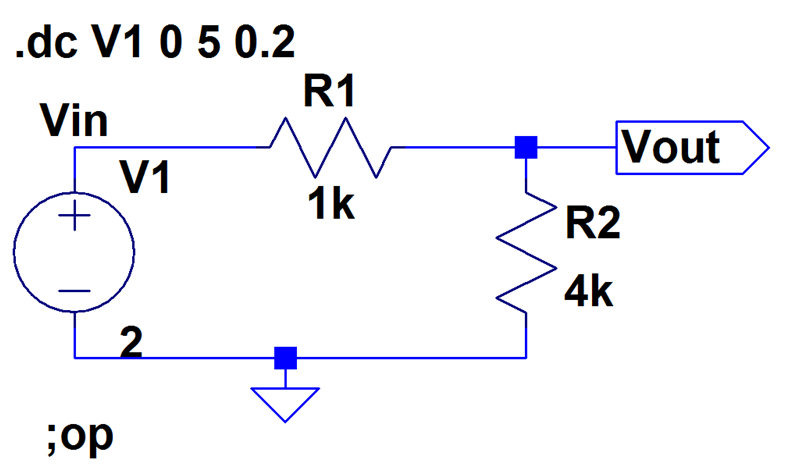Netlist * Spannungsteiler.asc V1 Vin 0 2 R1 Vout Vin 1k R2 Vout 0 4k ;op .dc V1 0 5 0.2 .backanno .end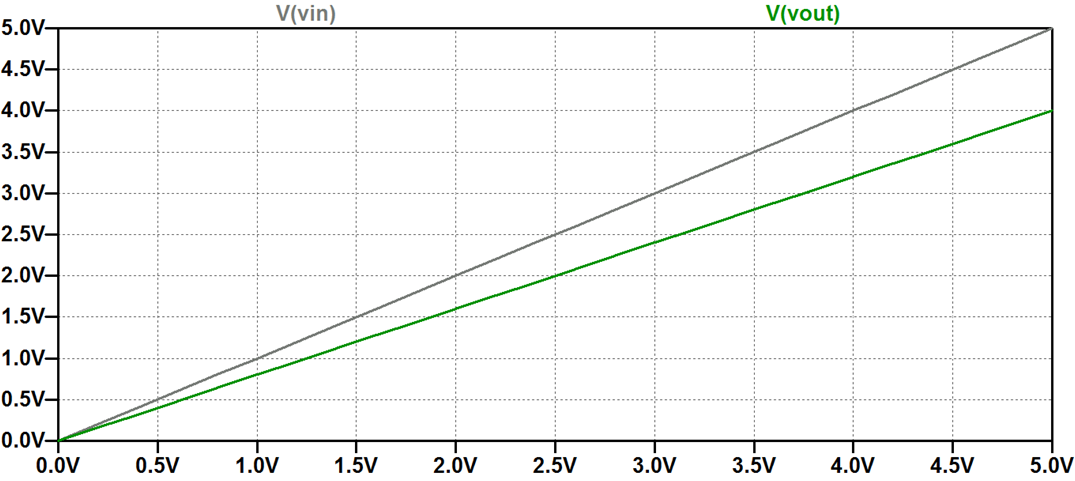# SPICE: Sinusodial source

 Right click on the source symbol and choose advanced SINE Simulate Edit Simulate Cmd .tran 0 2m 0m 0.01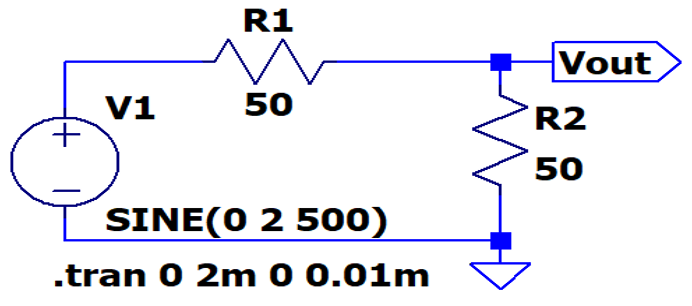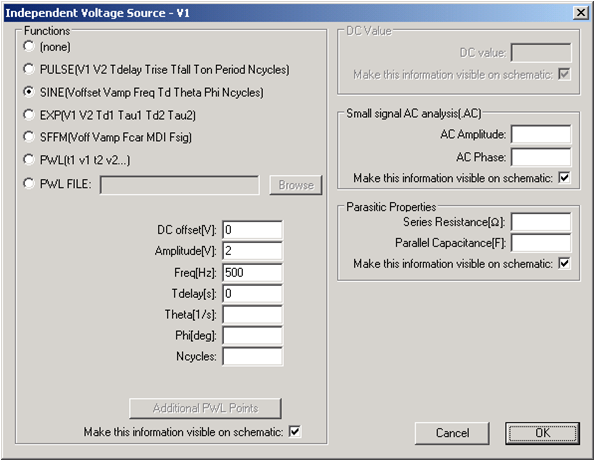# Transient simulation: .tran 1n 3u

 Schaltplan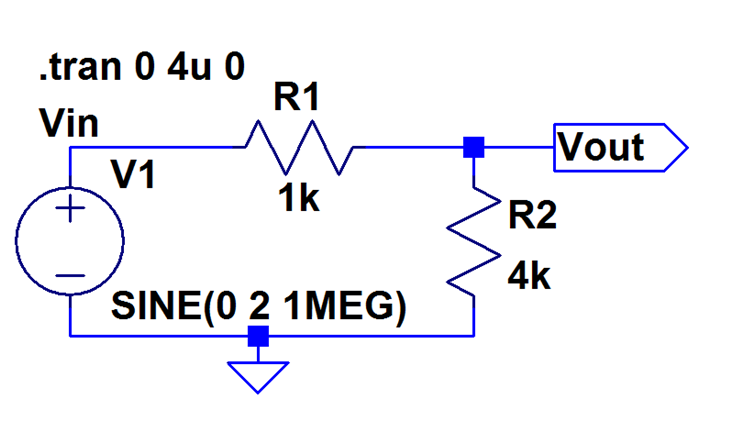* Spannungsteiler_02.asc V1 Vin 0 SINE(0 2 1MEG) R1 Vout Vin 1k R2 Vout 0 4k .tran 0 4u 0 .backanno .end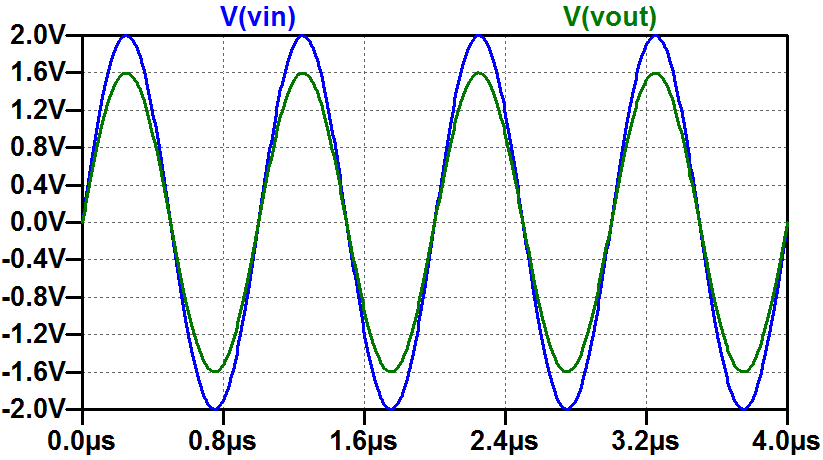# MOSFET transistors in LTSPICE

Netlist:
M1 NDrain NGate NSource PBulk NMOSModel
M2 PSource PGate PDrain PBulk PMOSModel
.model NX NMOS(LEVEL=1 KP=300u
+ VT0=0.8 LAMBDA=0.000 CGDO=400n)
.lib C:\Program Files (x86)\LTC\LTspiceIV\lib\cmp\standard.mos
.include cmosedu_models.txt
Example:
M1 VD VG VS VB N_50nm

The transistor model N_50nm can be specified in a model statement (.model), can be supplied in a library file (.lib) or in an external file (.include)
Symbol: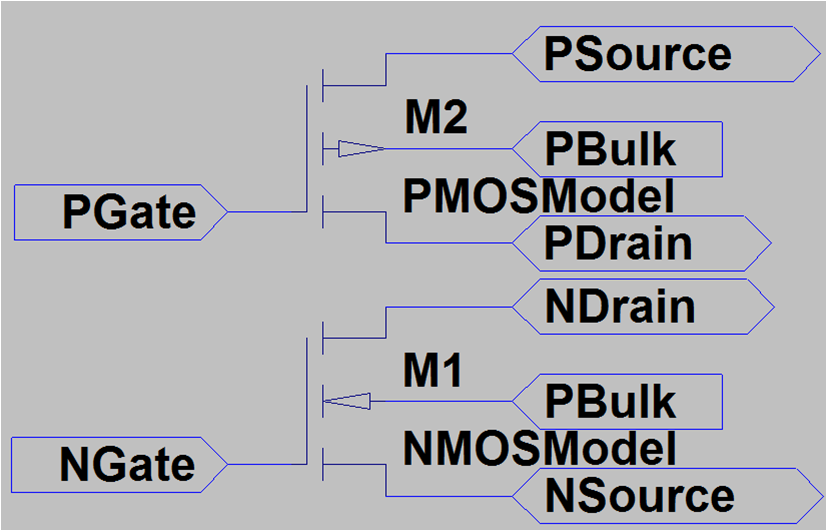# Transistor model

cmosedu_models.txt
 Simple model Detailed model .MODEL N_1u NMOS LEVEL = 3 + TOX = 200E-10 NSUB = 1E17 GAMMA = 0.5 + PHI = 0.7 VTO = 0.8 DELTA = 3.0 + UO = 650 ETA = 3.0E-6 THETA = 0.1 + KP = 120E-6 VMAX = 1E5 KAPPA = 0.3 + RSH = 0 NFS = 1E12 TPG = 1 + XJ = 500E-9 LD = 100E-9 + CGDO = 200E-12 CGSO = 200E-12 CGBO = 1E-10 + CJ = 400E-6 PB = 1 MJ = 0.5 + CJSW = 300E-12 MJSW = 0.5  .model N_50nm nmos level = 54 +binunit = 1 paramchk= 1 mobmod = 0 +capmod = 2 igcmod = 1 igbmod = 1 geomod = 1 +diomod = 1 rdsmod = 0 rbodymod= 1 rgatemod= 1 +permod = 1 acnqsmod= 0 trnqsmod= 0 +tnom = 27 toxe = 1.4e-009 toxp = 7e-010 toxm = 1.4e-009 +epsrox = 3.9 wint = 5e-009 lint = 1.2e-008 +ll = 0 wl = 0 lln = 1 wln = 1 +lw = 0 ww = 0 lwn = 1 wwn = 1 +lwl = 0 wwl = 0 xpart = 0 toxref = 1.4e-009 +vth0 = 0.22 k1 = 0.35 k2 = 0.05 k3 = 0 +k3b = 0 w0 = 2.5e-006 dvt0 = 2.8 dvt1 = 0.52 +dvt2 = -0.032 dvt0w = 0 dvt1w = 0 dvt2w = 0 +dsub = 2 minv = 0.05 voffl = 0 dvtp0 = 1e-007 +dvtp1 = 0.05 lpe0 = 5.75e-008 lpeb = 2.3e-010 xj = 2e-008 +ngate = 5e+020 ndep = 2.8e+018 nsd = 1e+020 phin = 0 +cdsc = 0.0002 cdscb = 0 cdscd = 0 cit = 0 +voff = -0.15 nfactor = 1.2 eta0 = 0.15 etab = 0 +vfb = -0.55 u0 = 0.032 ua = 1.6e-010 ub = 1.1e-017 +uc = -3e-011 vsat = 1.1e+005 a0 = 2 ags = 1e-020 +a1 = 0 a2 = 1 b0 = -1e-020 b1 = 0 +keta = 0.04 dwg = 0 dwb = 0 pclm = 0.18 +pdiblc1 = 0.028 pdiblc2 = 0.022 pdiblcb = -0.005 drout = 0.45 +pvag = 1e-020 delta = 0.01 pscbe1 = 8.14e+008 pscbe2 = 1e-007 +fprout = 0.2 pdits = 0.2 pditsd = 0.23 pditsl = 2.3e+006 +rsh = 3 rdsw = 150 rsw = 150 rdw = 150 +rdswmin = 0 rdwmin = 0 rswmin = 0 prwg = 0 +prwb = 6.8e-011 wr = 1 alpha0 = 0.074 alpha1 = 0.005 +beta0 = 30 agidl = 0.0002 bgidl = 2.1e+009 cgidl = 0.0002 +egidl = 0.8 +aigbacc = 0.012 bigbacc = 0.0028 cigbacc = 0.002 +nigbacc = 1 aigbinv = 0.014 bigbinv = 0.004 cigbinv = 0.004 +eigbinv = 1.1 nigbinv = 3 aigc = 0.017 bigc = 0.0028 +cigc = 0.002 aigsd = 0.017 bigsd = 0.0028 cigsd = 0.002 +nigc = 1 poxedge = 1 pigcd = 1 ntox = 1 +xrcrg1 = 12 xrcrg2 = 5 +cgso = 6.238e-010cgdo = 6.238e-010 cgbo = 2.56e-011 cgdl = 2.495e-10 +cgsl = 2.495e-10 ckappas = 0.02 ckappad = 0.02 acde = 1 +moin = 15 noff = 0.9 voffcv = 0.02 +kt1 = -0.21 kt1l = 0.0 kt2 = -0.042 ute = -1.5 +ua1 = 1e-009 ub1 = -3.5e-019 uc1 = 0 prt = 0 +at = 53000 +fnoimod = 1 tnoimod = 0 +jss = 0.0001 jsws = 1e-011 jswgs = 1e-010 njs = 1 +ijthsfwd= 0.01 ijthsrev= 0.001 bvs = 10 xjbvs = 1 +jsd = 0.0001 jswd = 1e-011 jswgd = 1e-010 njd = 1 +ijthdfwd= 0.01 ijthdrev= 0.001 bvd = 10 xjbvd = 1 +pbs = 1 cjs = 0.0005 mjs = 0.5 pbsws = 1 +cjsws = 5e-010 mjsws = 0.33 pbswgs = 1 cjswgs = 3e-010 +mjswgs = 0.33 pbd = 1 cjd = 0.0005 mjd = 0.5 +pbswd = 1 cjswd = 5e-010 mjswd = 0.33 pbswgd = 1 +cjswgd = 5e-010 mjswgd = 0.33 tpb = 0.005 tcj = 0.001 +tpbsw = 0.005 tcjsw = 0.001 tpbswg = 0.005 tcjswg = 0.001 +xtis = 3 xtid = 3 +dmcg = 0e-006 dmci = 0e-006 dmdg = 0e-006 dmcgt = 0e-007 +dwj = 0.0e-008 xgw = 0e-007 xgl = 0e-008 +rshg = 0.4 gbmin = 1e-010 rbpb = 5 rbpd = 15 +rbps = 15 rbdb = 15 rbsb = 15 ngcon = 1 

# Transistor measurement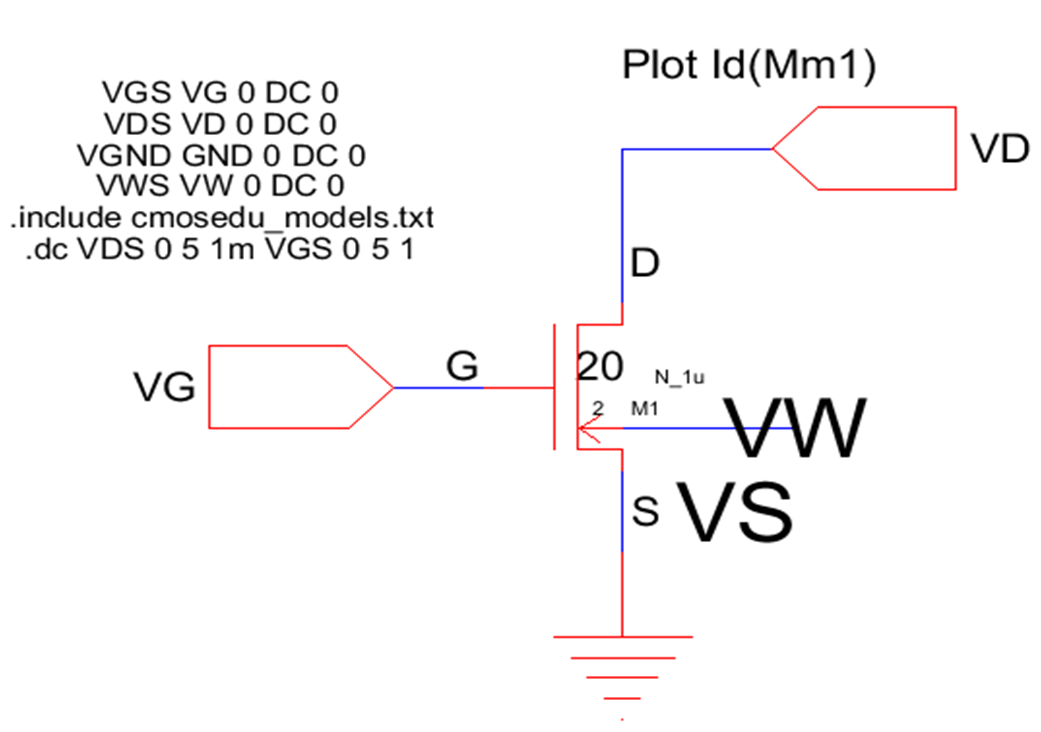# LTSPICE: Hierarchy

 Create a schematic with Pins: Edit->Label Net Port Type Create a symbol: preamp.asy for preamp.asc Edit->Add Pinport Make visible (TOP RIGHT BOTTOM LEFT) Place Instance Edit->Component Select correct directory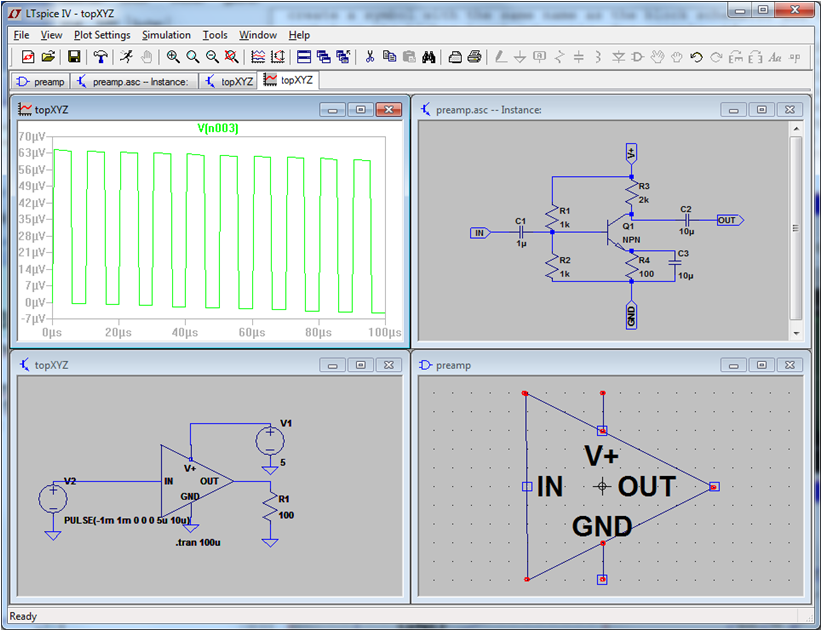# Hierarchy

SPICE directive in Netlist
.model CN NMOS(LEVEL=1 KP=11m VT0=1 LAMBDA=0.018 CGDO=400n)

Make a voltage node (VDD) available
.global VDD

Include device models or subcircuits
.include cmosedu_models.txt


A Circuit can be reused
Nodes to be connected externally need to be set as input and output: label net
A symbol needs to be created: Hierarchy, Open this Sheets symbol
Insert this circuit using Edit, Components and select a local directory to make locally defined cirucits visible.

Use of a subcircuit is shown starting with X in netlist. Definition starts with .subckt and ends with ends name.

       XX1 IN D7 IN1 pipestageinv

* block symbol definitions
.subckt pipestageinv In Dout Vout
M1 N001 In 0 0 CN
…
ends pipestageinv


# Input waveforms and measurement

 Waveforms VA A 0 PWL file=a.txt VB B 0 PWL file=B.txt VC Ci 0 PWL file=C.txt a.txt 0n 0 9n 0 10n 1 19n 0 20n 1 29n 1 30n 0 Calculation of average and rms value Select the legend of a curve in the Waveformwindow. < strg > Mouse left click Measurement of time: .Measure TAX00 WHEN V(ax)=0.5 FALL=1 .Measure TY101 WHEN V(y1)=0.5 RISE=1 .Measure DY101 PARAM (TY101-TAX00)*1E12 Result name: TAX00 Time when voltage of node ax is 0.5V and ax is the first falling edge.

# Hilfe LTSPICE für Temperaturvariation: Suchbegriff R, TEMP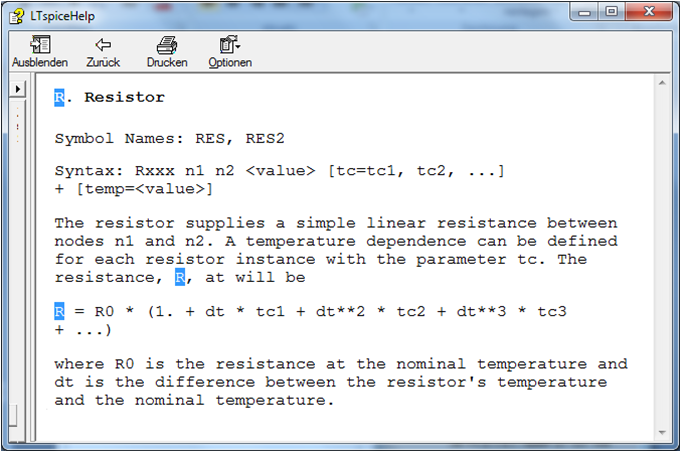# Simulation with varying components

 create a parameter with curly brackets. {R2} Set a value .param R2 100 change the value: .step parameter .step parameter R2 100 300 100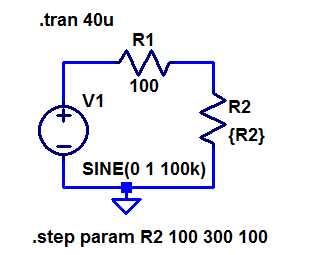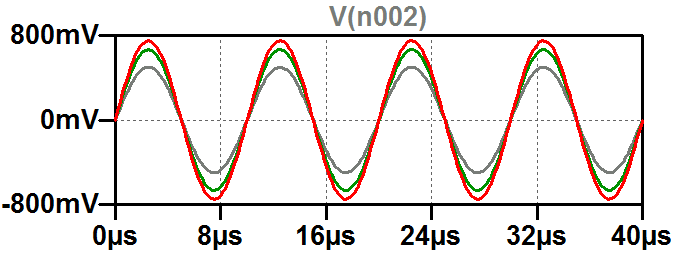# Behavioral data converter simulation

• Behavioral simulation: voltage controlled voltage sources (bv)
Simple mathematical equation
• Inputs and Outputs:
Data D0..DN-1, Vin or Vout
time discrete: clock, sample and hold (switch and capacitance)
• Time simulation:
Ramp or sine
All possible codes
FFT: number of samples power of 2: 2N+4
+4 for accuracy and sine function.
Sine signal prime number of periods

Example: 20 Bit ADC, fs = fclk = 100 MHz, Range 0..1 V, Vref = 1V
Simulation time: tsim = 2 20 + 4 /100MHz = 16M/100M s = 0.16777216s
Signal sine frequency: fsig = prime / tsim

# Behavioral data converter simulation

 Bits LSB Simulation time: tsim signal frequency:fsig LTSPICE run time LTSPICE output file size FFT points Comment 20 1 μ V 167.77216ms 65.56510925 Hz 167.7ms/1.2us/s = 100 000s = 30 h 2MB/us => 600 GB minimal; 4600s => 6ms => 7.8GB 16 → 98 dB 16 μ V 220 * 10 ns = 10.48576 ms $\frac{11}{t_{sim}} = 1049.04174804687 Hz$ 8467s = 2.5 h 900 MB $2^{20} = 1048576$ → 57dB LTSPICE can't draw curve, but can load data and do FFT 12 256 μ V 216 * 10 ns = 655.36 µs $\frac{11}{t_{sim}} = 16784.66796875 Hz$ 378s =7 min 65 MB $2^{16} = 65536$ 8 4 → 26 dB 62.5 mV 28 * 10 ns = 2.560 µ s $\frac{11}{t_{sim}} = 4296875 Hz$ 1.4 s 500 kB $2^{8} = 256$ →

.save dialog
.save V(in) V(d*) V(Vout)
This saves only V(in), all data channels and Vout and reduces the file size significantly.

; option can limit saved data
.option numdgt=12
precision of saved data

To be able to use simulated data for FFT with high precision use the following option:
.options plotwinsize=0

# Scalable behavioral 4 Bit DAC

 Behavioral voltage source BV with equation V=V(in1)/16+V(D3)/2+V(D2)/4+V(D1)/8+V(D0)/16 Scalable DAC takes input signal In1 as high resolution input and adds D3..D0 information. For high resolution more modules can be combined.

# Scalable behavioral 4 Bit ADC

 Behavioral voltage source BV Rounding function: V(D3) = round(V(IN)) V(D2) = round(V(IN)*2-V(D3)) ... Residue: V(Out) = V(in)*16-V(D3)*8-V(D2)*4-V(D1)*2-V(D0) Residue allows extending the ADC for high resolution.

# Test for 4 Bit ADC and DAC

 A 4 Bit ADC and DAC test can be simulated in LTSPICE. The output file size can be limited by using the .save dialog option. The output shows the step size of the digitalisation.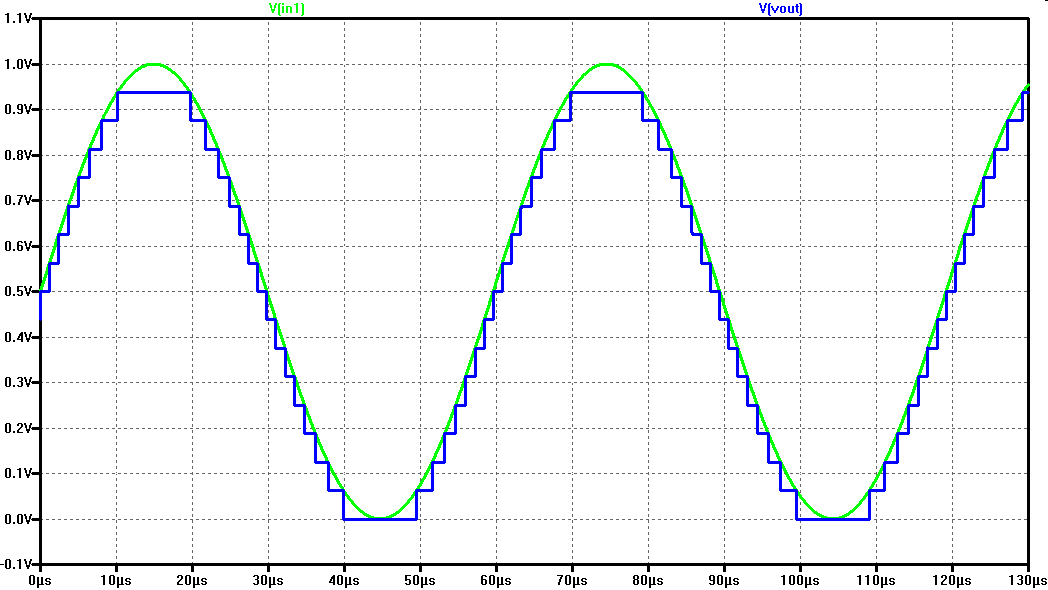# Test for 12 Bit ADC and DAC

 A 12 Bit ADC and DAC test can still be simulated in LTSPICE. The output file size can be limited by using the .save dialog option. FFT 65k points gives: signal: -9dB, noise level: -116dB Calculation: 6.07 * B dB + 1.76 dB + 10 log(N/2) dB = 6.07 * 12 dB + 1.76 dB + 10 log(65k/2) dB = 72 dB + 1.76 dB + 45 dB = 119 dB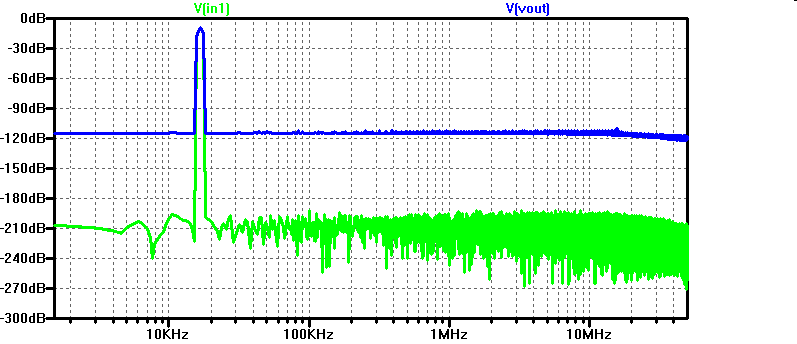Analyzing this data with Read LTSPICE raw file for data converter analysis. with Start = 0, Stop = 655.36E-6, Step 10E-6 and procesing integer values with FFT and INL, DNL data converter analysis gives you less SQNR than expected due to sample and hold circuits distroting the residue.

A comparison of the signals res0 and vout0, res1 and vout1 shows the performance of the circuit. The signals should be identical.

It is unclear why the FFT in LTSPICE is not showing this.

# Improved Test for 12 Bit ADC and DAC

 A 12 Bit ADC and DAC test can still be simulated in LTSPICE. The output file size can be limited by using the .save dialog option. Sample and hold circuits are only used at the input and output. Javascript FFT 16k points gives: signal: 84.3dB, noise level: 8.56dB Calculation: 6.07 * B dB + 1.76 dB + 10 log(N/2) dB = 6.07 * 12 dB + 1.76 dB + 10 log(16k/2) dB = 72 dB + 1.76 dB + 40 dB = 119 dB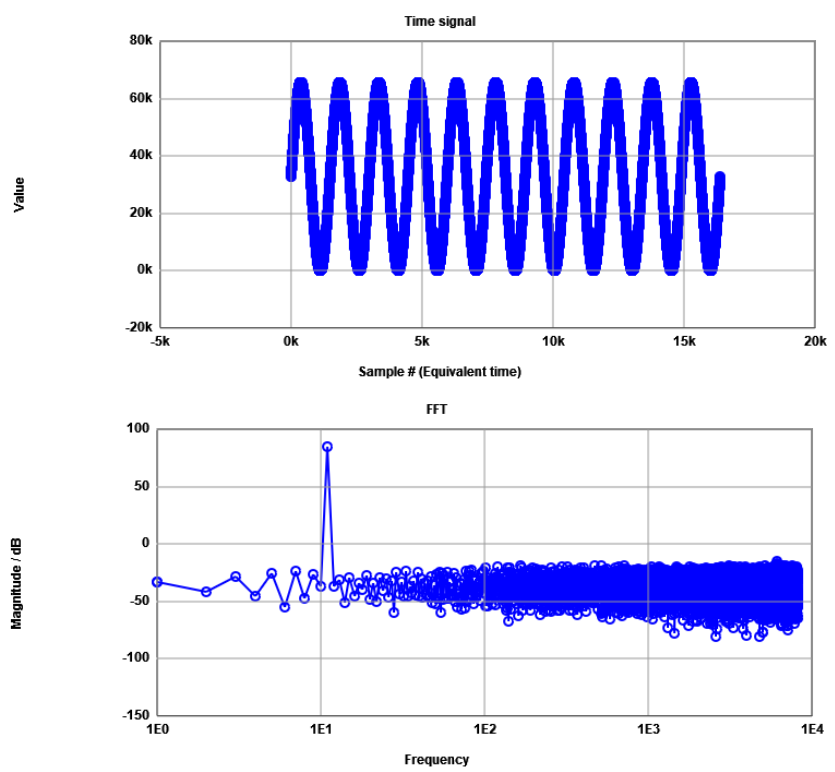Start time 0, Stop time 655.36E-6, Time step 40E-9 gives 16384 data points.
Mapping this with a scale of 65535 to integer prepares analysis with Javascript FFT.

# Test data analysis

### LTSPICE raw data file size reduction

LTSPICE data can be reduced with a .save statement.

### Reading LTSPICE raw data via a web page

Read LTSPICE raw file for data converter analysis.

### Data converter analysis via a web page

FFT and INL, DNL data converter analysis.

There is an option to do calibration before.

### LTSPICE raw data file size reduction

LTSPICE data can be reduced with a .save statement.
.save V(vout)
The simulation of the 12-bit ideal ADC and DAC gives for 11 periods sine simulation running 5 min in LTSPICE generating 4 million points and 48 MB file size.
This file can be loaded into Matlab with a LTspice2Matlab code (watch out for UTF8 versus UTF16 encoding).

Adapt lines in the code accordingly:
153 - fid = fopen(filename, 'rb', 'n', 'UTF16LE');
156 - fid = fopen(sprintf( '%s.raw', filename ), 'rb', 'n', 'UTF16LE');

It can also be processed on a web page:
Read LTSPICE raw file for data converter analysis.
Before reading the data choose a time step of 10E-9 to get data for each conversion step.
Choose a range of at least 65536 to get at least 16 bit for further processing.
After browsing for the file it takes quite some time, a couple of minutes, in Firefox until data is loaded and converter.
More then 65k points are generated.

### Data converter analysis

Another web page will do the analysis:
FFT and INL, DNL data converter analysis.
First data is copied to the text input field.
Then read integer data is done.
Finishing with Generate charts.
FFT, INL and DNL are displayed. Further down on the page signal to noise is displayed.